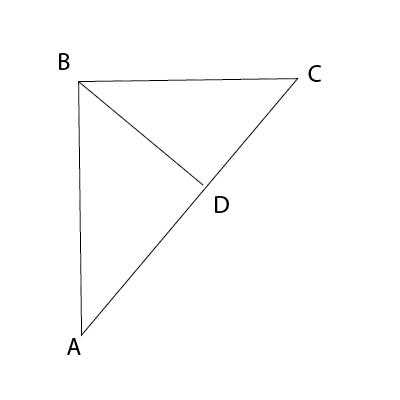### CAT 1999 Question Paper Question 49

Instructions

DIRECTIONS for the following three questions

These questions are based on the situation given below:

A road network (shown in the figure below) connects cities A, B, C and D. All road segments are straight lines. D is the midpoint on the road connecting A and C. Roads AB and BC are at right angles to each other with BC shorter than AB. The segment AB is 100 km long. Ms. X and Mr. Y leave A at 8:00 am, take different routes to city C and reach at the same time. X takes the highway from A to B to C and travels at an average speed of 61.875 km per hour. Y takes the direct route AC and travels at 45 km per hour on segment AD. Y's speed on segment DC is 55 km per hour.Question 49

# The total distance traveled by Y during the journey is approximately

Solution

$$BC^2$$ + 10000 = $$AC^2$$.

$$\frac{BC+100}{61.875}$$ = $$\frac{AC}{49.5}$$ => $$\frac{BC+100}{AC}$$ = 1.25 = > BC = 1.25AC - 100

On solving these equations, we get AC as 105 and BC a 31.

=> Y traveled 105 km.